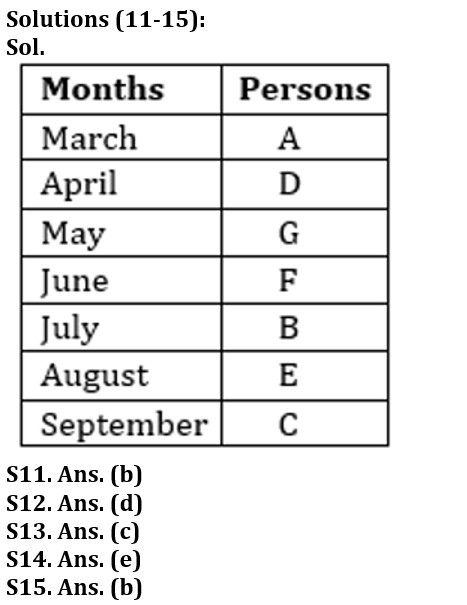Latest Banking jobs   »

# Reasoning Ability Quiz For LIC AAO 2023- 27th January

Directions (1-5): Study the following digit-letter-symbol sequence carefully and answer the questions given below:

C % B K 1 & A W 5 P E 4 Q @ 7 F 6 G © Z J L 2 € D ¥ M # 8

Q1. How many such symbols are there in the above sequence, each of which is immediately preceded by a consonant and immediately followed by a number?
(a) None
(b) One
(c) Two
(d) Four
(e) None of these

Q2. Which of the following is fifth to the left of 15th element from the left?
(a) S
(b) P
(c) E
(d) #
(e) None of these

Q3. If all the numbers are dropped in the given series, then which element will be at sixth position from right end?
(a) Z
(b) M
(c) L
(d) D
(e) None of these

Q4. How many such numbers are there in the above sequence, each of which is immediately preceded by a vowel and immediately followed by a consonant?
(a) Three
(b) Two
(c) None
(d) One
(e) None of these

Q5. What should come in place of question mark in the following on the basis of above sequence?
B, &, P, 7, ?
(a) #
(b) M
(c) D
(d) 2
(e) J

Direction (6-10): Study the following alphanumeric series carefully and answer the questions given below:

S * 7 B \$ © G 2 8 U 1 & A V # F 3 5 H @ 5 Y 5 M 3 2 D 8 % N 2 4

Q6. How many such numbers are there which is preceded by alphabets and followed by symbols?
(a) One
(b) Two
(c) Three
(d) Four
(e) None

Q7. Which of the following element is 19th from right end of the series?
(a) #
(b) F
(c) 3
(d) V
(e) None of these

Q8. How many such symbols are there which is preceded by numbers and followed by vowels?
(a) Two
(b) None
(c) One
(d) Four
(e) Three

Q9. How many such alphabets are there which is preceded and followed by same number?
(a) Three
(b) Two
(c) One
(d) Four
(e) None

Q10. If all numbers are eliminated from the series, then, which of the following element is 11th from right end of the series?
(a) V
(b) A
(c) &
(d) #
(e) None of these

Directions (11-15): Study the information carefully and answer the questions given below:
Seven persons are going to participate in quiz contest in seven different months starting from March to September. D goes in the month which have even numbers of days. More than three persons are going in between D and C. Only two persons going in between F and C. E goes after G, who does not go in March. Three persons are going in between A and B. A does not go after C. More than one-month gap between A and F going for the quiz.

Q11. Who among the following person goes in April?
(a) E
(b) D
(c) A
(d) B
(e) None of these

Q12. Who among the following person are going immediate after the month in which E goes?
(a) B
(b) D
(c) G
(d) C
(e) None of these

Q13. Four of the following are alike in a certain way so form a group, which among the following does not belong to that group?
(a) E
(b) A
(c) F
(d) B
(e) None of these

Q14. Which of the following statement is true about C?
(a) C goes before F
(b) Only three persons go between C and G
(c) G goes just before C
(d) C goes in September
(e) Both (b) and (d)

Q15. How many persons are going in between G and B?
(a) Two
(b) One
(c) Three
(d) More than Three
(e) None

Solutions

S1. Ans.(c)
Sol. Q@7, M#8

S2. Ans. (b)
S3. Ans. (c)

S4. Ans. (d)
Sol. E4Q
S5. Ans. (e)

S6. Ans. (b)
Sol. U1&, D8%

S7. Ans. (d)

S8. Ans. (c)
Sol. 1&A

S9. Ans. (c)
Sol. 5 Y 5

S10. Ans. (b)## FAQs

### When will the LIC AAO Prelims exam be held?

The LIC AAO Prelims exam will be held on 17th & 20th of February 2023 (tentatively).

#### Congratulations!Union Budget 2023-24: Free PDF